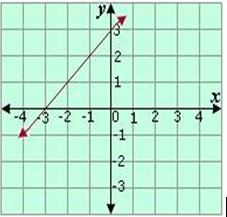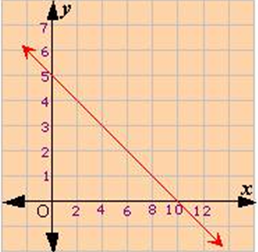## Definition Of X-Intercept

The x-intercept of a line is the point at which the line crosses the x-axis.

The y-coordinate of the point where a line intersects the x-axis is 0
So, the x-intercept of a line can also be found by substituting y = 0 in the equation of the line.

### Examples of X-InterceptAs the line shown in the graph is intersecting the x-axis at the point (-3, 0), so the x-intercept of the line is -3.

### Solved Example on X-Intercept

#### Ques: Find the x-intercept of the graph of 4x + 8y = 40.

##### Choices:

A. - 5
B. 10
C. 5
D. - 10